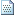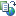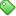PHP教程

# Javascript中定义类

今天工作需要，又要封装一些类，总觉得还有些东西理解的不够，今天看了下面文章，感觉透彻了，分享给大家。

Javascript本身并不支持面向对象，它没有访问控制符，它没有定义类的关键字class，它没有支持继承的extend或冒号，它也没有用来支持虚函数的virtual，不过，Javascript是一门灵活的语言，下面我们就看看没有关键字class的Javascript如何实现类定义，并创建对象。

function Shape()
{
var x = 1 ;
var y = 2 ;
}

var aShape = new Shape();

aShape.x = 1 ;

function Shape()
{
this .x = 1 ;
this .y = 2 ;
}

aShape.x = 2 ;

function Shape()
{
var x = 0 ;
var y = 1 ;
this .draw = function ()
{
// print;
};
}

aShape.draw();

function Shape()
{
var x = 0 ;
var y = 1 ;
var draw = function ()
{
// print;
};
}

Javascript并不支持OOP，当然也就没有构造函数了，不过，我们可以自己模拟一个构造函数，让对象被创建时自动调用，代码如下：
function Shape()
{
var init = function ()
{
// 构造函数代码
};

init();
}

function Shape(ax,ay)
{
var x = 0 ;
var y = 0 ;
var init = function ()
{
// 构造函数
x = ax;
y
= ay;
};

init();
}

var aShape = new Shape( 0 , 1 );

function Shape(ax,ay)
{
var x = 0 ;
var y = 0 ;
var init = function ()
{
// 构造函数
x = ax;
y
= ay;
};

init();
}
Shape.count
= 0 ; // 定义一个静态属性count，这个属性是属于类的，不是属于对象的。
Shape.staticMethod = function (){}; // 定义一个静态的方法

aShape.staticMethod();

function Shape(ax,ay)
{
var x = 0 ;
var y = 0 ;
this .gx = 0 ;
this .gy = 0 ;
var init = function ()
{
x
= ax; // 访问私有属性，直接写变量名即可
y = ay;
this .gx = ax; // 访问公有属性，需要在变量名前加上this.
this .gy = ay;
};

init();
}

this指针指错的场合一般在事件处理上面，我们想让某个对象的成员函数来响应某个事件，当事件被触发以后，系统会调用我们这个成员函数，但是，传入的this指针已经不是我们本身的对象了，当然，这时再在成员函数中调用this当然会出错了。

function Shape(ax,ay)
{
var _this = this // 把this保存下来，以后用_this代替this，这样就不会被this弄晕了
var x = 0 ;
var y = 0 ;
_this.gx
= 0 ;
_this.gy
= 0 ;
var init = function ()
{
x
= ax; // 访问私有属性，直接写变量名即可
y = ay;
_this.gx
= ax; // 访问公有属性，需要在变量名前加上this.
_this.gy = ay;
};

init();
}文章来自: 本站原创Tags: